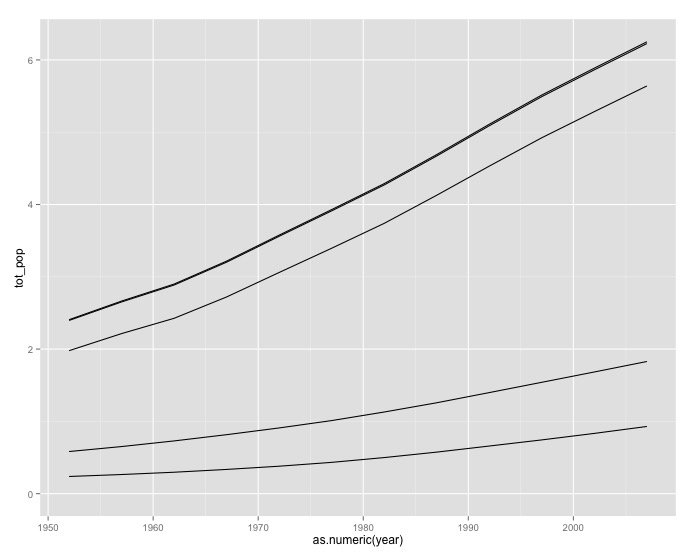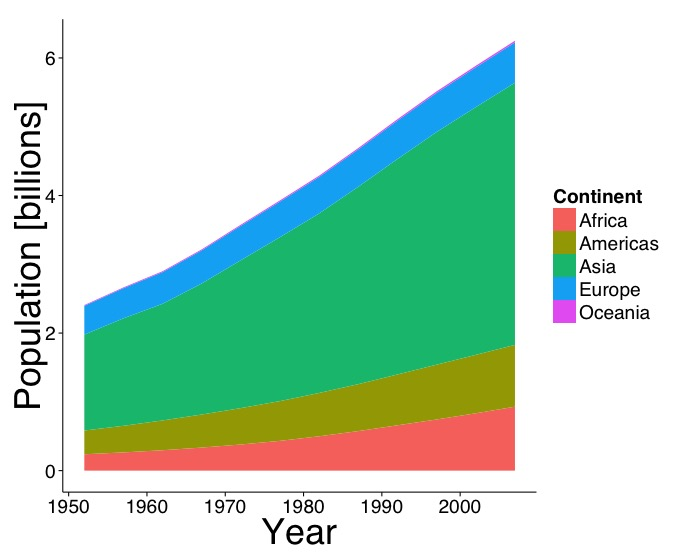# Stacked Area Chart

## Overview

Teaching: 5 min
Exercises: 15 min
Questions
• What kind of scientific question does a stacked area chart address?

• How do I generate a stacked area chart with `ggplot2`?

Objectives
• To generate a stacked area chart using `ggplot2`

Let students walk through decision making steps from question to chart.

• “How has the composition of the world population among continents changed from 1952-2007?”
• Composition changing over time
• Stacked area chart

Implement faceted table of histograms.

• `geom_line()`, `geom_area()`
• `guide_legend()`

## Chart Improvement Challenge

Here is a incomplete (or bad) example of the chart we are going for. Modify the code to a publication quality stacked area chart.

``````total_pop_continent<- pop_by_continent %>%
group_by(continent, year) %>%
summarize(tot_pop = sum(population)/1000000000)

ggplot(total_pop_continent, aes(as.numeric(year), tot_pop,
group = continent)) +
geom_line(position = "stack")
``````## Output Solution## Code Solution

``````ggplot(total_pop_continent, aes(as.numeric(year), tot_pop,
fill = continent)) +
geom_area() +
labs(x = "Year", y = "Population [billions]",
fill = "Continent") +
theme_classic(base_size = 24, base_family = "Helvetica") +
theme(axis.title = element_text(size = 36))
``````

## Key Points

• Stacked area charts represent a composition changing over time.